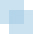# Growth Models

##INTRODUCTION

In this appendix, general expressions for estimating growth rates are presented.

• Compound annual growth (CAGR) rate model
• General model for estimating CFaIAg, the growth rate of Cash Flow after Investing Activities (CFaIA), and NIg, the growth rate of Net Income (NI), from the Investment Rate (IR) and Net Income Return on Capital Employed (NiROCE)

##GENERAL COMPOUND ANNUAL GROWTH RATE MODEL

Let VBeg and VEnd represent the beginning and ending values of a series of numbers that develop over n periods. Then VEnd can be expressed in terms of VBeg and n by Equation [C-1].where: CAGR is the compound annual growth rate1

Taking logarithms of both sides of Equation [C-1],Rearranging:Multiplying both sides of [C-3] by n−1Since n−1 (n) = 1 and, Equation ...

Get Corporate Value Creation: An Operations Framework for Nonfinancial Managers now with the O’Reilly learning platform.

O’Reilly members experience books, live events, courses curated by job role, and more from O’Reilly and nearly 200 top publishers.